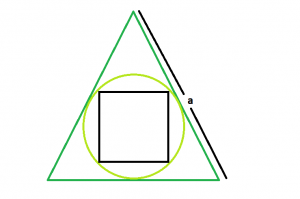# Area of a square inscribed in a circle which is inscribed in an equilateral triangle

Given here is an equilateral triangle with side length a, which inscribes a circle, which in turn inscribes a square. The task is to find the area of this square.

Examples:

```Input: a = 6
Output: 1

Input: a = 10
Output: 0.527046

```

## Recommended: Please try your approach on {IDE} first, before moving on to the solution.Approach:

let r be the radius of circle,
hence it is the inradius of equilateral triangle, so r = a /(2 * √3)
diagonal of square, d = diameter of circle = 2 * r = a/ √3
So, area of square, A = 0.5 * d * d
hence A = (1/2) * (a^2) / (3) = (a^2/6)

Below is the implementation of the above approach:

## C++

 `// C++ Program to find the area of the square ` `// inscribed within the circle which in turn ` `// is inscribed in an equilateral triangle ` `#include ` `using` `namespace` `std; ` ` `  `// Function to find the area of the square ` `float` `area(``float` `a) ` `{ ` ` `  `    ``// a cannot be negative ` `    ``if` `(a < 0) ` `        ``return` `-1; ` ` `  `    ``// area of the square ` `    ``float` `area = ``sqrt``(a) / 6; ` ` `  `    ``return` `area; ` `} ` ` `  `// Driver code ` `int` `main() ` `{ ` `    ``float` `a = 10; ` `    ``cout << area(a) << endl; ` `    ``return` `0; ` `} `

## Java

 `// Java Program to find the area of the square ` `// inscribed within the circle which in turn ` `// is inscribed in an equilateral triangle ` ` `  `import` `java.io.*; ` ` `  `class` `GFG { ` `    `  ` `  `// Function to find the area of the square ` `static` `float` `area(``float` `a) ` `{ ` ` `  `    ``// a cannot be negative ` `    ``if` `(a < ``0``) ` `        ``return` `-``1``; ` ` `  `    ``// area of the square ` `    ``float` `area = (``float``)Math.sqrt(a) / ``6``; ` ` `  `    ``return` `area; ` `} ` ` `  `// Driver code ` `    ``public` `static` `void` `main (String[] args) { ` `        ``float` `a = ``10``; ` `    ``System.out.println( area(a)); ` `// This code is contributed  ` `// by  inder_verma.. ` `    ``} ` `} `

## Python 3

 `# Python3 Program to find the area  ` `# of the square inscribed within   ` `# the circle which in turn is  ` `# inscribed in an equilateral triangle  ` ` `  `# import everything from math lib. ` `from` `math ``import` `*` ` `  `# Function to find the area  ` `# of the square  ` `def` `area(a): ` ` `  `    ``# a cannot be negative  ` `    ``if` `a < ``0` `: ` `        ``return` `-``1` ` `  `    ``# area of the square  ` `    ``area ``=` `sqrt(a) ``/` `6` ` `  `    ``return` `area ` ` `  `# Driver code      ` `if` `__name__ ``=``=` `"__main__"` `: ` ` `  `    ``a ``=` `10` `    ``print``(``round``(area(a), ``6``)) ` ` `  `# This code is contributed by ANKITRAI1 `

## C#

 `// C# Program to find the area  ` `// of the square inscribed within  ` `// the circle which in turn is  ` `// inscribed in an equilateral triangle ` `using` `System; ` ` `  `class` `GFG  ` `{ ` `     `  `// Function to find the area  ` `// of the square ` `static` `float` `area(``float` `a) ` `{ ` ` `  `    ``// a cannot be negative ` `    ``if` `(a < 0) ` `        ``return` `-1; ` ` `  `    ``// area of the square ` `    ``float` `area = (``float``)Math.Sqrt(a) / 6; ` ` `  `    ``return` `area; ` `} ` ` `  `// Driver code ` `public` `static` `void` `Main () ` `{ ` `    ``float` `a = 10; ` `    ``Console.WriteLine(area(a)); ` `} ` `} ` ` `  `// This code is contributed  ` `// by inder_verma `

## PHP

 ` `

Output:

```0.527046
```

Don’t stop now and take your learning to the next level. Learn all the important concepts of Data Structures and Algorithms with the help of the most trusted course: DSA Self Paced. Become industry ready at a student-friendly price.

My Personal Notes arrow_drop_upCheck out this Author's contributed articles.

If you like GeeksforGeeks and would like to contribute, you can also write an article using contribute.geeksforgeeks.org or mail your article to contribute@geeksforgeeks.org. See your article appearing on the GeeksforGeeks main page and help other Geeks.

Please Improve this article if you find anything incorrect by clicking on the "Improve Article" button below.

Improved By : inderDuMCA, AnkitRai01